# Four NOR gates are connected as shown in figureQuestion:

Four NOR gates are connected as shown in figure.

The truth table for the given figure is :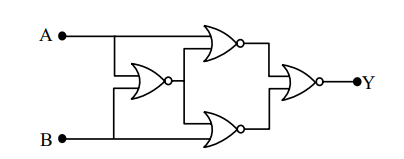1.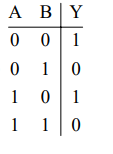2.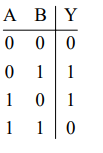3.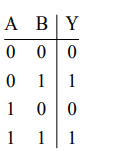4.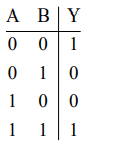Correct Option: , 4

Solution: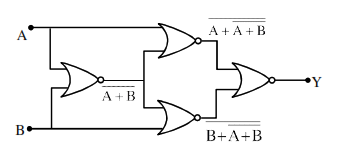$y=\overline{(\overline{A+\overline{A+B}})+(\overline{B+\overline{A+B})}}$

$\mathrm{y}=(\mathrm{A}+\overline{\mathrm{A}+\mathrm{B}}) \cdot(\mathrm{B}+\overline{\mathrm{A}+\mathrm{B}})$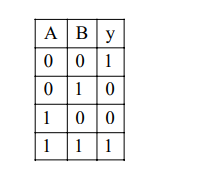Ans. 4